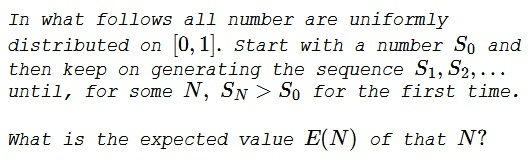# Waiting for a Larger Number

### Problem### Solution 1

Talking of probabilities, we disregard the possibility of getting two equal number, as having zero probability. Further,

$P(N\gt n)=P(S_0\gt S_1,S_0\gt S_2,\ldots,S_0\gt S_n)$

because, from the definition of $N,$ none of the previous numbers exceeded $S_0.$ Put another way,

$P(N\gt n)=P(max\{S_0,S_1,S_2,\ldots,S_n\}=S_0).$

Now, here's a crucial observation: all $n+1$ numbers are uniformly distributed on $[0,1]$ so there is nothing special about $S_0$ and, in principle, any of them could be the largest. Thus $P(N\gt n)=\displaystyle\frac{1}{n+1}.$ (This is an instance of invocation of the Principle of Symmetry.) Similarly, $P(N\gt n-1)=\displaystyle\frac{1}{n}.$ Now,

$\displaystyle P(N=n)=P(N\gt n-1)-P(N\gt n)=\frac{1}{n}-\frac{1}{n+1}=\frac{1}{n(n+1)}.$

To continue,

$\displaystyle E(N)=\sum_{n=1}^{\infty}n\cdot\frac{1}{n(n+1)}=\sum_{n=1}^{\infty}\frac{1}{n+1}=\infty,$

strange as it may appear.

On one hand, $P(N\gt n)=\displaystyle\frac{1}{n+1}$ indicates that not finding a maximum after, say, $99$ attempts, has a fairly low probability of $\displaystyle \frac{1}{100}.$ On the other hand, the expected waiting interval for that to happen is infinite.

### Solution 2

By symmetry, $E[N]$ is unchanged if we stop instead when $S_N \lt S_0.$ For any given $S_0,$ this takes $\displaystyle\frac{1}{S_0}$ tries on average, so $\displaystyle E[N] = \frac{1}{S_0}.$ Finally, since $S_0$ is uniformly distributed over $[0,1],$ we have

$\displaystyle E[N] = (\text{area under the curve }\frac{1}{S_0}\text{ for }0 \le S_0 \le 1) = \infty.$

### Solution 3

Integral of $(1-x)(1+2x+3x^2+\ldots)$ from $0$ to $1$ is what it is. The second term is just the derivative of $1+x+x^2+\ldots$ with respect to $\displaystyle x = \frac{1}{(1-x)^2}.$ The integral of $\displaystyle \frac{1}{1-x}$ from $0$ to $1$ diverges.

### Solution 4

The probability of first exit on $1^{st}$ shot $P(S_1\gt S_0)=1-S_0.$

The probability of first exit on $2^{nd}$ shot $P(S_1\lt S_0,S_2\gt S_0)=S_0(1-S_0).$

The probability of first exit on $t^{th}$ shot $P(S_1\lt S_0,\ldots,S_{t-1}\lt S_0,S_t\gt S_0)=S_0^{t-1}(1-S_0).$

The conditional stopping time (knowing $S_0),$ is

$\displaystyle E(N|S_0)=\sum_{t=1}^{\infty}tS_0^{t-1}(1-S_0)=\frac{1}{1-S_0}.$

The unconditional stopping time (not knowing $S_0),$ is

$\displaystyle \lim_{H\to 1}\lim_{L\to 0}\int_{L}^{H}\frac{dx}{1-x}=\lim_{H\to 1}\lim_{L\to 0}\ln\left(\frac{1-L}{1-H}\right)=\infty.$

### Acknowledgment

The problem is a rephrase of one from P. J. Nahin's Dueling Idiots and Other Probability Puzzles (Princeton University Press, 2000, 31-32).

Solution 1 is an adaptation of Nahin's solution; Solution 2 is by Josh Jordan; Solution 3 is by Amit Itagi, Marcos Carreira and several others; Solution 4 is by N. N. Taleb.Copyright © 1996-2018 Alexander Bogomolny

 65250385